# Blockchain and cryptography （区块链与密码学）学习笔记3：群与公钥加密

Hacker Dōjo Workshop：

Bounty链接：https://dorahacks.io/zh/daobounty/139

Telegram: @HackerDojo0
WeChat: @HackerDojo0
E-mail: hackerdojo0@gmail.com

# 3 群与公钥加密

## 3.1 群

1. 非空集： 集合中至少有一个元素。

2. 二元运算： 集合中的元素能够进行一种运算，例如加法运算、或乘法运算。

3. 封闭性： 集合中的元素进行运算后，得到的结果仍然是集合中的元素。

4. 结合律： 任意a,b,c属于G，则(a+b)+c=a+(b+c)。

5. 单位元e 加法情况下a+e=e+a=a，乘法情况下：ae=ea=a。

6. 每个元素 都有逆元a^(-1) ：加法情况下a+ a^(-1)= a^(-1)+a=e，乘法情况下：a*a^(-1)= a^(-1)*a=e。

1. 非空集： 群内有7个元素。

2. 二元运算: 加法。

3. 封闭性： 群内任意两个元素相加后模7后仍然是群中的元素，例如(5+6)mod7=4；

4. 结合律： ((3+4)+5) mod7=(3+(4+5)) mod7=5，结果相同。

5. 单位元为e=0 3+0=0+3=3。

6. 逆元： 1的逆元为6，因为(1+6)mod7=e，2的逆元为5，因为(2+5)mod7=e，同理3的逆元为4。0的逆元是0。

7 是素数，这个素数群的性质特别好。因为素数7 与群元素i 是互素的，所以每个非零元素都是群的生成元 例如：群元素2能够通过有限次运算，表达其他所有元素：

(2+2)mod7=4则表达群元素4，

(2+2+2)mod7=6则表达群元素6，

(2+2+2+2)mod7=1则表达群元素1，

(2+2+2+2+2)mod7=3则表达群元素3，

(2+2+2+2+2+2)mod7=5则表达群元素5，

(2+2+2+2+2+2+2)mod7=0则表达群元素0。

(3)mod7=3

(3+3)mod7=6

(3+3+3)mod7=2

(3+3+3+3)mod7=5

(3+3+3+3+3)mod7=1

(3+3+3+3+3+3)mod7=4

(3+3+3+3+3+3+3)mod7=0

(4)mod7=4

(4+4)mod7=1

(4+4+4)mod7=5

(4+4+4+4)mod7=2

(4+4+4+4+4)mod7=6

(4+4+4+4+4+4)mod7=3

(4+4+4+4+4+4+4)mod7=0

(5)mod7=5

(5+5)mod7=3

(5+5+5)mod7=1

(5+5+5+5)mod7=6

(5+5+5+5+5)mod7=4

(5+5+5+5+5+5)mod7=2

(5+5+5+5+5+5+5)mod7=0

(6)mod7=6

(6+6)mod7=5

(6+6+6)mod7=4

(6+6+6+6)mod7=3

(6+6+6+6+6)mod7=2

(6+6+6+6+6+6)mod7=1

(6+6+6+6+6+6+6)mod7=0

1. 非空集： 群内有6个元素。

2. 二元运算: 乘法

3. 封闭性： 群内任意两个元素相乘后模7后仍然是群中的元素，例如(5*6)mod7=2；

4. 结合律： ((34) 5) mod7=(3 (45)) mod7=4，结果相同。

5. 单位元为1 1乘以任意元素等于任意元素;31=13=3。

6. 逆元： 1的逆元为1，因为(11)mod7=1;2的逆元为4，因为(24)mod7=e=1; 3的逆元为5，因为(35)mod7=1。6的逆元为6，因为(66)mod7=1。

(2)mod7=2

(2*2)mod7=4

(222)mod7=1

(222*2)mod7=2

(22222)mod7=4

(22222*2)mod7=1

(2222222)mod7=2

(3)mod7=3，记为31mod7=3

(3*3)mod7=2，记为32mod7=2

(333)mod7=6，记为33mod7=6

(333*3)mod7=4，记为34mod7=4

(33333)mod7=5，记为35mod7=5

(33333*3)mod7=1，记为36mod7=1

``````         (3*3*3*3*3)mod7=5，记为3<sup>5</sup>mod7=5
``````

## 3.2 Diffie-Hellman密钥交换

Alice私钥SK1= α，公钥PK1=gα

Bob私钥SK2=β，公钥PK2=gβ;

Bob发送其公钥给Alice。

Alice私钥SK1= α=4,公钥PK1=gα=24mod11=5;
Bob私钥SK2= β=5,公钥PK2=gβ=25mod11=10;

(PK1)SK2=55mod11=1

Bob计算如下(PK1SK2=(gα)β=gαβ，令Key=Hash（gαβ，r1，r2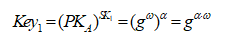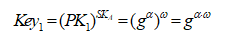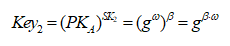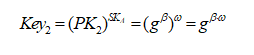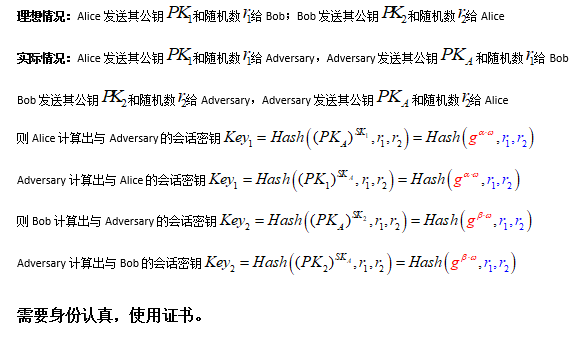## 3.3 ElGamal加密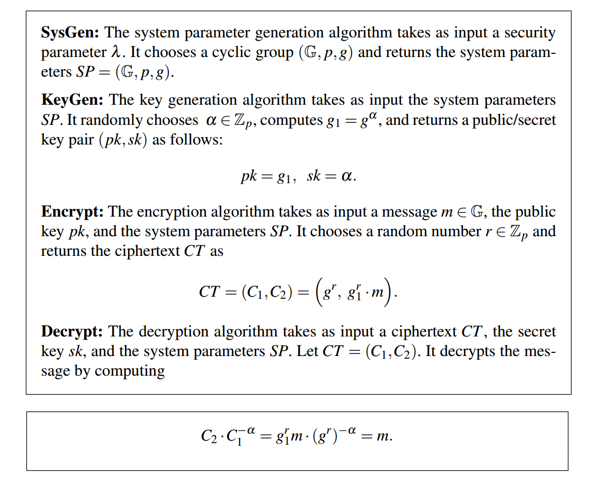## 3.4 椭圆曲线群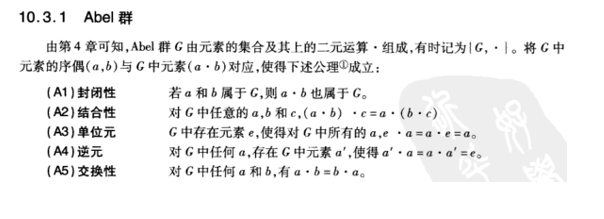## 3.6 ElGamal同态加密

### 3.6.1 密文同态运算原理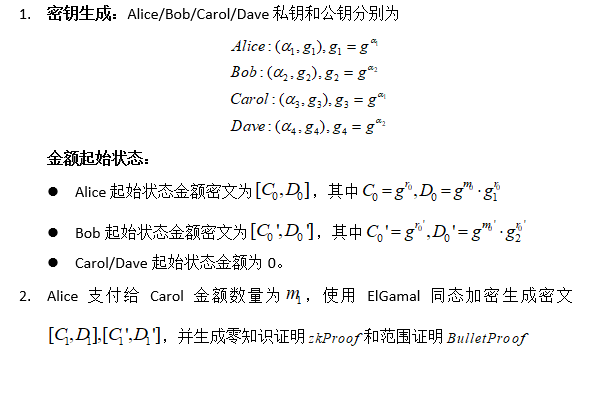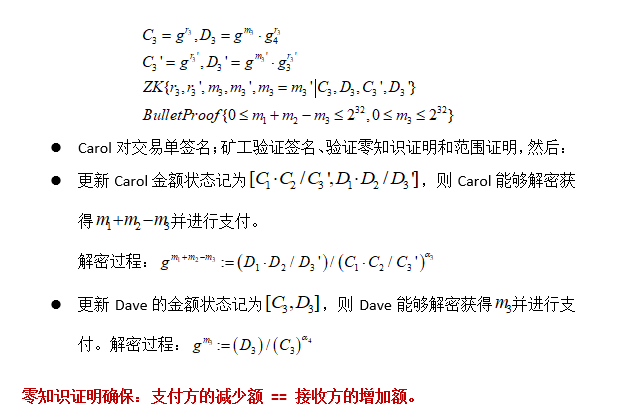## 3.8 ECIES加密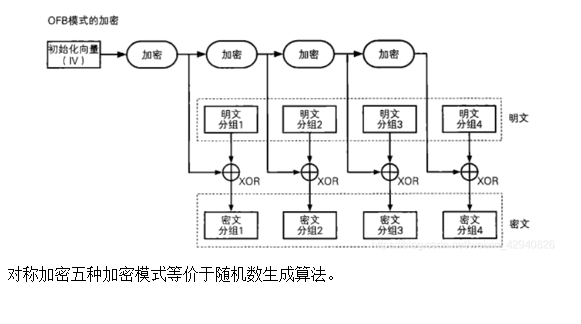Hacker Dōjo是由Hacker共建的加密、Web3前沿技术开源知识社区。Dōjo会以直播/音频/文字等形式定期组织分享session，内容包括Web3领域前沿技术论文解读、技术研讨、工作坊等。欢迎在Hacker Dōjo社区讨论、学习和交流。加入Dōjo的Hacker可以提出自己的学习期望，主动提案自己擅长的技术话题，由Dōjo组织分享。同时，Hacker Dōjo推出Web3前沿课题研究计划，定期选题，由hacker进行研究和讲解，并以bounty形式奖励研究贡献者。认领Bounty：DoraHacks转载文章：请保留Hacker Dōjo Workshop介绍并联系我们hackerdojo0@gmail.com

## 3.3 ElGamal加密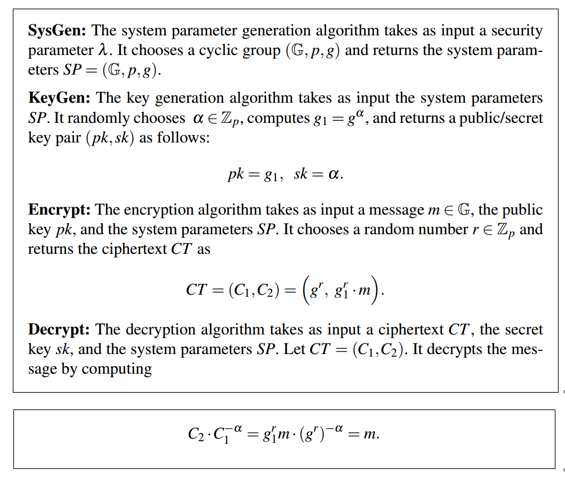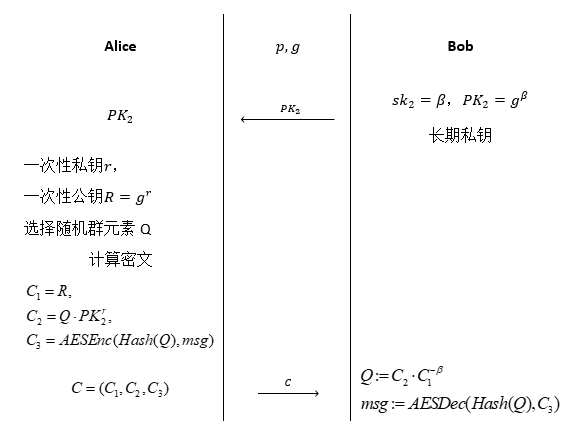## 3.4 椭圆曲线群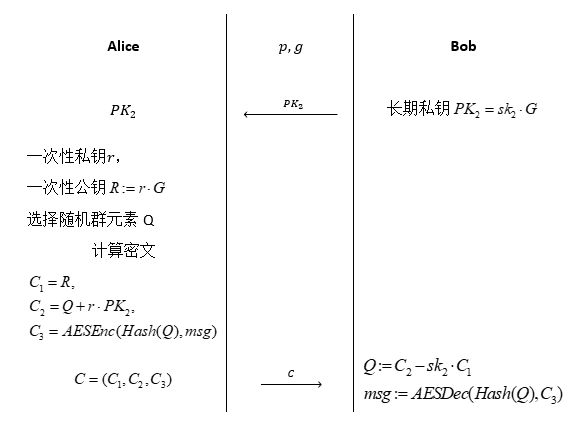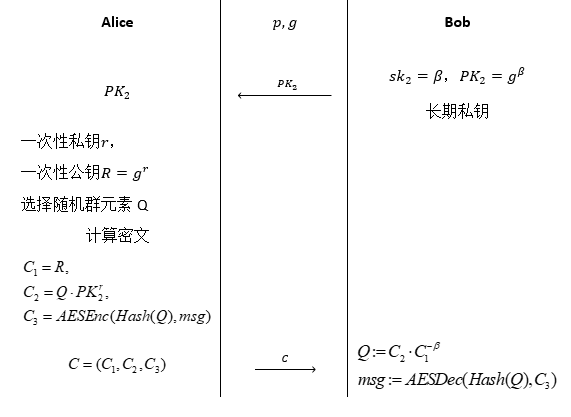Good job! Very useful!